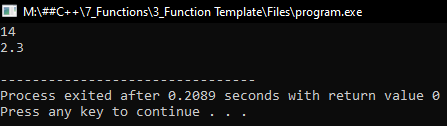# Function Template in C++

## Function Template in C++ with Examples:

##### What are Function Templates in C++?

Function templates are the functions that are generic. Generic in the sense, it’s generalized in terms of data type.

```#include<iostream>
using namespace std;
int Max(int x, int y)
{
if (x > y)
return x;
else
return y;
}

float Max(float x, float y)
{
if (x > y)
return x;
else
return y;
}

int main()
{
cout << Max(10, 5) << endl;
cout << Max(12.5f, 13.4f) << endl;
return 0;
}
```

To understand generic functions i.e. template functions, we have taken two functions here with the same name Max(). They are the same but the data type of the parameters of one function is ‘int’ and another is of ‘float’ type. So, they are overloaded functions. The number of parameters is the same but the data type of parameters is different.

From the main function, depending on the parameters we will call the functions. If our data is in ‘float’ values then ‘float Max()’ will be called and If our data is in ‘int’ values then ‘int Max()’ will be called.

Now the thing here is, we can see the body of these functions or the logic written is the same, only the difference is in the data type. Logic is the same. So why write the same function two times when there is only a difference in the data type. So, can’t we write a single function combining these two functions for any data type any type of data? Yes, we can write.

##### How to write Function Template in C++?

Please have a look at the below code.

template<class T>;
T Max(T x, T y){
if(x > y)
return x;
else
return y;
}

Here T means template. We have defined a template class ‘T’, so ‘template <class T>’ is a definition of ‘T’ which is defined as a class of type template. So, the function is a template function. We have written the same logic but instead of data type ‘int’ or ‘float’ we have replaced them with ‘T’. Now let us write the main function.

int main(){
int c = Max(10, 5);
float d = Max(10.5f, 6.9f);
}

Here when we are calling ‘Max(10, 5)’ then ‘T’ becomes ‘int’ as we are passing ‘int’ values and when we are calling ‘Max(10.5f, 6.9f)’ then ‘T’ becomes ‘float’ as we are passing ‘float’ values. So, the same function work as a ‘float’ as well as ‘int’.

The benefit of the function template is that we can write down multiple functions together as a single function where there is a difference in the data type only.

This template function will not just work for ‘int’ or ‘float’, but it works for any data type we pass or even if we pass the objects of classes. This works perfectly for any type of data. This is one of the most powerful features of C++ and this is a confusing feature also if you don’t understand it you cannot use it properly.

This is a more programmer-friendly feature where this feature was removed in other languages like Java and C# but later, they have introduced to some extent but this is full in the control of programmer in C++. Let us see the complete program.

##### Program to understand Function Template in C++:
```#include<iostream>
using namespace std;

template <class T>
T Max(T a, T b)
{
return a > b ? a : b;
}

int main()
{
cout << Max (12, 14) << endl;
cout << Max (2.3, 1.4) << endl;
return 0;
}
```
###### Output:#### Function Template – Frequently Asked Questions

##### max() function is giving error

The max() is an inbuilt function in C++. Change the name to maxim()

No.

##### class vs type name

Both are the same. You can use anyone

##### Can we initialize the template variable?

Yes. It should be initialized only with 0.